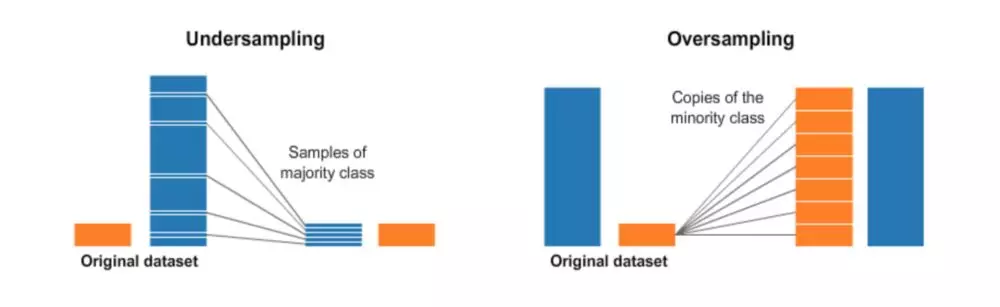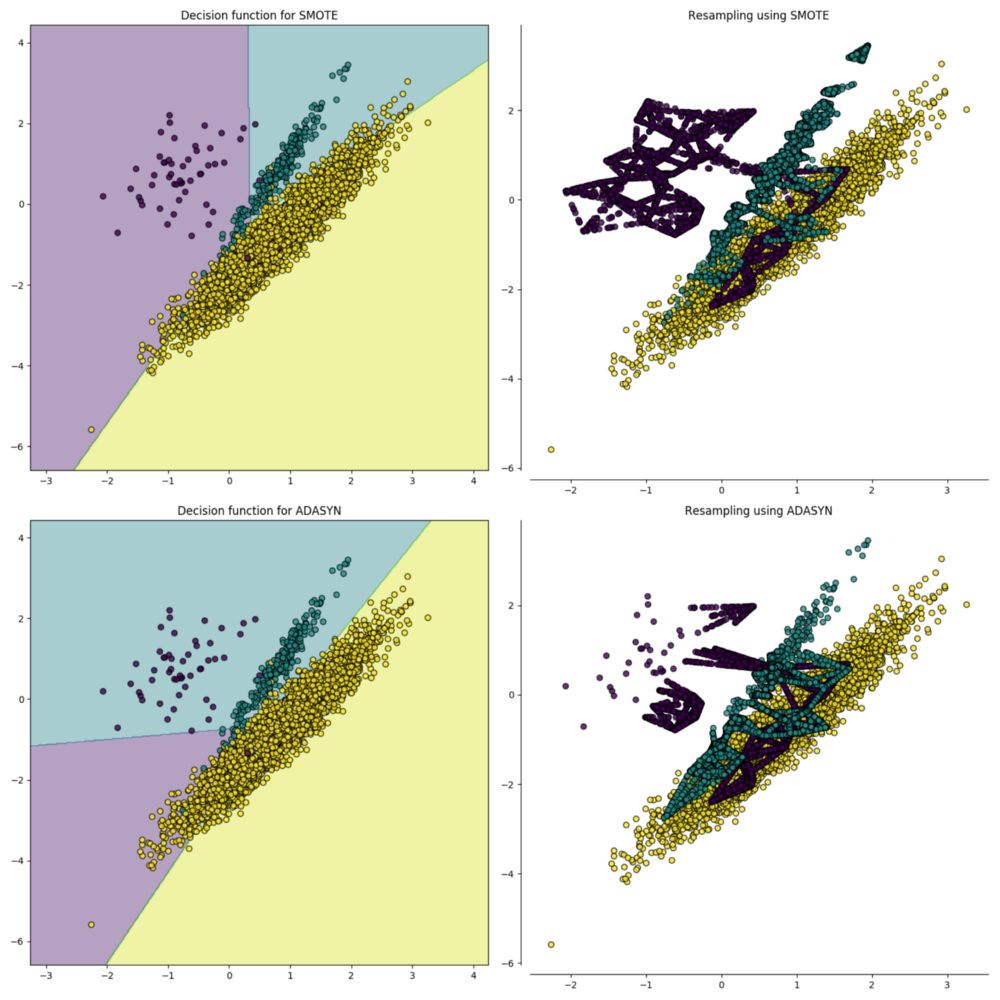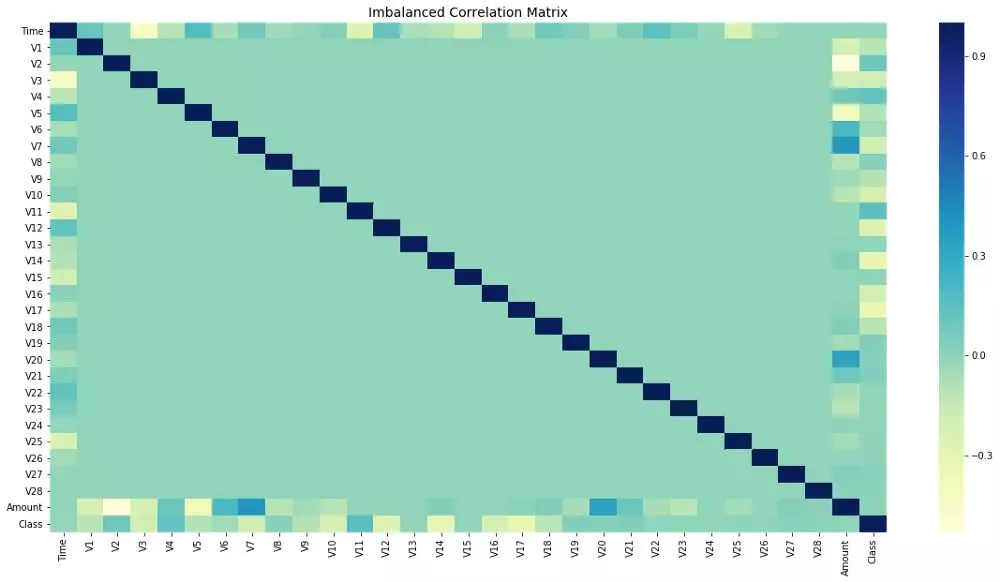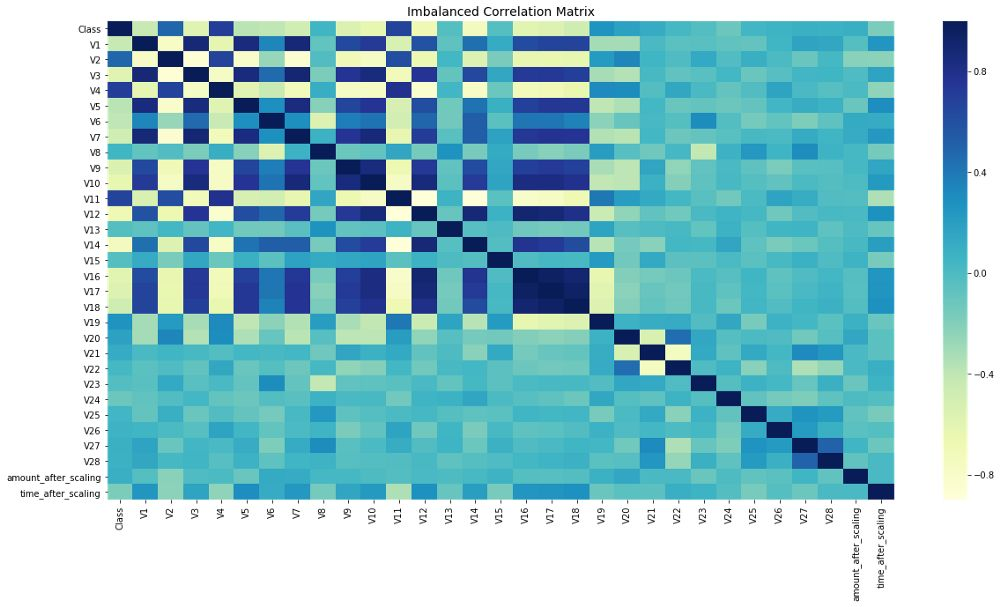独家 | 一文教你如何处理不平衡数据集（附代码）

+关注继续查看

什么是数据不平衡（类别不平衡）？

https://www.kaggle.com/mlg-ulb/creditcardfraudhttps://github.com/wmlba/innovate2019/blob/master/Credit_Card_Fraud_Detection.ipynb

一、 重采样（过采样和欠采样）# Shuffle the Dataset.

shuffled_df = credit_df.sample(frac=1,random_state=4)

# Put all the fraud class in a separate dataset.

fraud_df = shuffled_df.loc[shuffled_df['Class'] == 1]

#Randomly select 492 observations from the non-fraud (majority class)

non_fraud_df=shuffled_df.loc[shuffled_df['Class']== 0].sample(n=492,random_state=42)

# Concatenate both dataframes again

normalized_df = pd.concat([fraud_df, non_fraud_df])

#plot the dataset after the undersampling

plt.figure(figsize=(8, 8))

sns.countplot('Class', data=normalized_df)

plt.title('Balanced Classes')

plt.show()来源

imbalanced-learn

from imblearn.over_sampling import SMOTE

# Resample the minority class. You can change the strategy to 'auto' if you are not sure.

sm = SMOTE(sampling_strategy='minority', random_state=7)

# Fit the model to generate the data.

oversampled_trainX,oversampled_trainY=sm.fit_sample(credit_df.drop('Class', axis=1), credit_df['Class'])

oversampled_train=pd.concat([pd.DataFrame(oversampled_trainY), pd.DataFrame(oversampled_trainX)], axis=1)

oversampled_train.columns = normalized_df.columns

# Sample figsize in inches

fig, ax = plt.subplots(figsize=(20,10))

# Imbalanced DataFrame Correlation

corr = credit_df.corr()

sns.heatmap(corr, cmap='YlGnBu', annot_kws={'size':30}, ax=ax)

ax.set_title("Imbalanced Correlation Matrix", fontsize=14)

plt.show()https://towardsdatascience.com/why-feature-correlation-matters-a-lot-847e8ba439c4

二、 集成方法（采样器集成）

BalancedBaggingClassifier

https://mp.weixin.qq.com/cgi-bin/appmsg?t=media/appmsg_edit&action=edit&type=10&isMul=1&isNew=1&lang=zh_CN&token=89565677#imblearn.ensemble.BalancedBaggingClassifier

from imblearn.ensemble import BalancedBaggingClassifier

from sklearn.tree import DecisionTreeClassifier

#Create an object of the classifier.

bbc = BalancedBaggingClassifier(base_estimator=DecisionTreeClassifier(),

sampling_strategy='auto',

replacement=False,

random_state=0)

y_train = credit_df['Class']

X_train = credit_df.drop(['Class'], axis=1, inplace=False)

#Train the classifier.

bbc.fit(X_train, y_train)

preds = bbc.predict(X_train)

How to fix an Unbalanced Dataset

https://www.kdnuggets.com/2019/05/fix-unbalanced-dataset.html

译者简介ICPR 2018｜阿里巴巴读光OCR及MTWI数据集亮相引关注
2276 01622 0【许晓笛】49行代码就能发币？而且EOS连例子都给你了
1056 01065 0【3万患者11万图像14类病理】NIH公开大规模胸部X光数据集
4507 0《深入解析sas：数据处理、分析优化与商业应用》一3.2　操作数据集的观测
2139 01950 0SAS学习笔记之《SAS编程与数据挖掘商业案例》（3）变量操作、观测值操作、SAS数据集管理
SAS学习笔记之《SAS编程与数据挖掘商业案例》（3）变量操作、观测值操作、SAS数据集管理 1. SAS变量操作的常用语句 ASSIGNMENT 创建或修改变量 SUM 累加变量或表达式 KEEP 规定在数据集中保留的变量 DROP 规定在数据集中删除的变量 ARRAY 定义一个数组 RENAME
1431 0Amazon如何利用云服务处理大规模多维数据集
1285 0SAS进阶《深入解析SAS》之对多数据集的处理
SAS进阶《深入解析SAS》之对多数据集的处理 1. 数据集的纵向串接： 数据集的纵向串接指的是，将两个或者多个数据集首尾相连，形成一个新的数据集。 据集的横向合并： 数据集的横向合并，指的是将两个或者多个数据集根据某种原则横向合并起来，形成新的数据集。 2. 数据集的纵向串接两种方法：1）使用SAS DATA步的SET语句。2）使用SAS过程步的APPEND过程。
1264 0
770

584

+ 订阅

《2021云上架构与运维峰会演讲合集》

《零基础CSS入门教程》

《零基础HTML入门教程》